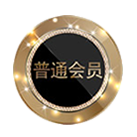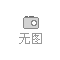## 青岛海晨希纺织有限公司# 生产服装服饰针织经编带.黑白现货.横拉带厂家直销

## 诚信档案

### 企业名片

【友情提示】：来电请说明在发布每一天看到我们的，谢谢！

## 商品信息

≥10000 米

10000 米

#### 有效期至：

rrr 专业批发服装服饰针织经编带，黑白色现货，横拉带规格齐全，经编带厂家直销！
r
r

rr 友情提示：经编带，100米/把，整把米数起拍。 r

rrr

rrrrrrrrrrrrr
r
r

rr 郑重声明 r

rr 本公司是正规生产厂家，价格均是大货出厂价。不像所谓的中间商或贸易商，价格不真实。用质量，米数说话，是公司一直以来不变的宗旨。 r

rr   r

rr 快速了解 r

rr r r r r r r r r r r r r r r r r r r r r r r r r r r r r
 r r 品名： r r r r 经编带 r r r r 材料： r r r r 低弹丝r r r r r 型号： r r r r JBD-HCX r r r r 品牌： r r r r 海晨希 r r r r 产地： r r r r 青岛即墨 r r r r 颜色： r r r r 黑/白 r r r r 用途： r r r r 多种服装，服饰，帽绳，帽子产品r r r r r 规格： r r r r 10mm  12mm  15mm  20mm r r
rr   r

rr 关于产品 r

rr 1：材质 r

rr 纱线均采用国内ISO认证的环保低弹丝，这样不仅保证了生产的长期稳定性，让客户放心大胆的使用不会产生后顾之忧。 r

rr 2：包装 r

rr 现货经编带为方便管理及销售，都是采用打把模式（50米/把，100米/把），不同规格下的包装数量，参照表格 r

rr r r r r r r r r r r r r r r r r r r r r r r r r r r r r r r r r r r r r r r r r r r r r
 r r 规格 r r r mm r r r r 每袋数量 r r r /把 r r r r 每包数量 r r r /米 r r r r 每把数量 r r r /米 r r r r 备注 r r r r 10 r r r r 100 r r r r 10000 r r r r 100 r r r r   r r r r 12 r r r r 100 r r r r 10000 r r r r 100 r r r r   r r r r 15 r r r r 100 r r r r 10000 r r r r 100 r r r r   r r r r 20 r r r 100 r r 5000 r r 50 r r r r r   r r r r   r r r r   r r r r   r r
rr 3：用途 r

rr 服装，服饰，鞋帽，玩具，家纺等，广泛运用在多行业。 r

rr
r

rr 4：宽度 r

rr
r

rr 原白客户多用于染色，一般出机宽度比实际宽度要多出1毫米左右。 r

rr
r

rr 5：运费 r

rr 轻松下单，付款后客服给你安排发货。大货批发价，不包邮的，若是量大，咨询包邮问题可联系在线客服。
r r

rr
r

rr
r

rr 订购指南 r

rr
r

rr （1）现货供应 外用经编带，黑/白不同品质，多种规格，长年均有现货。轻松下单，快乐你我。我们的备货，解决了客户因订单量少，采购难的问题。 r

rr
r

rr （2）特殊定做 若您遇到单量大，但现货品质不能满足您需求的时候，不用担心。你可以联系我们的在线客服，然后把您的样品寄过来。这边按照您的样品进行分析核价。相信优惠的价格及高效的打样，能给你带来方便。 r

rr
r

rr （3）专属设计 高端化的外用经编带可以让您的产品得到更高的评价。您可以提供图纸及思路，让我们的专业团队帮您去设计开发。新思路，新层次，新发展。 r

rr
r

rr
r

rr 温馨提示 r

rr
r

rr 经编带自身有弹性，受生产制约，宽度上下会有1mm的误差，属于纺织行业的正常允许范围。但根本不影响使用，介意的请慎拍。 r

rr
r

rr   r

rr 发货流程 r

rr 经编带品质分多种，可以按照自己所需要的品质，选择使用的数量提交订单，待付款成功后会有客服安排统一发出。如果下单时不明白的地方，可以随时咨询我们的在线客服，帮您解答。 r

rr
r

rr 退货须知 r

rr 经编带网上图片都是实物拍摄，但受外界环境及光线的影响，难免会与现货有小小的色差，但品质是没问题的。因此理由退货或者想更换其他品质及规格的，只要货物无损，厂家可以给处理，往返运费自理。 r

rr
r

rr 公司简介  r

rr      青岛海晨希纺织有限公司，坐落于美丽的海滨城市青岛，是一家织带与印花相结合的纺织企业。 r

rr      公司成立于2002年，建筑面积5000平方米，现有员工60余人。多年来，公司不断更新设备，引进一流的生产技术，聘请顶级的研发人员。现拥有无梭机50台、钩编机20台，经编机10台，印花机3台、绳带机120台。日产织带50万米，年销售额2000万元。 r

rr      生产印花带，提花带，经编带，松紧带、绳类产品。织带印花方式有机器丝网、手工印花、热转印、硅胶印花等。自有专业的印花生产线，在保证印花质量的基础上，大大缩短了客户的交期。松紧带、经编带，背包带及绳类产品，常规品质，多种规格，常年均备有大量现货。 r

rr      产品被广泛运用于服装、箱包、鞋帽、工艺品、玩具，电子，汽车、展会等行业。先后为阿迪、FILA、长春一汽、北京现代等多家知名的公司提供织带，建立了长期合作关系。产品远销韩国、日本、欧美等国家。 r

rr      多年以来，公司始终奉行“服务为上，价格保优，不断进取”的经营理念，坚守“保质，保量，守时”的生产原则，相信我们《研发，生产，销售》为一体的服务团队，能给你带来意想不到的便利。 r

rr   r

rr
r

rr 联系方式 r

rr 联系人：裴女士 r

rr 电话：14753232769 r

rr
r

rr
r

rr   r

rr
r

0相关评论

## 相关栏目

### 按排行字母分类：

A B C D E F G H I J K L M N O P Q R S T U V W X Y Z